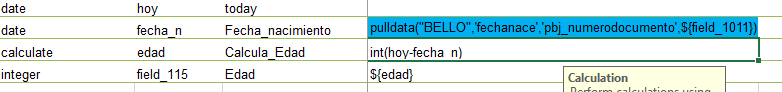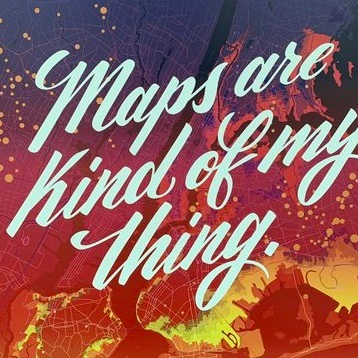# Age calculation

690
3
08-15-2019 07:22 AMNew Contributor III

how to subtract two dates to calculate the ageI have this survey, there is the question of today's date, then I bring the date of birth, but by subtracting both dates in the next question it makes me wrong.

Tags (4)
3 RepliesbyMVP Regular Contributor

Ismael has an example in his blog post: https://community.esri.com/groups/survey123/blog/2016/04/17/dates-and-time-in-survey123-for-arcgis

EPOCH FormatDecimal Date Format
Calculate age from birthdayint((today() - \${birth_date}) div (1000*24*60*60* 365.25))int((decimal-date-time(today()) - decimal-date-time(\${birth_date})) div 365.25)

Also, it looks like you're missing the proper \${fieldname} notation in your calculation.

You can use today() in the calculation to automatically get today's date without having to use another question in your surveyNew Contributor II

Greetings,

This was quite helpful. Decimal Date format works with both connect and the web as opposed to the EPOCH Format which appears to work with the Field app only. Question: how can you do a calculation for the age of a baby in months? I was able to get the calculation to work in years but what formula can I use to calculate the age of a baby in months?byMVP Regular Contributor

If you multiple it by 12, you will get months:

((decimal-date-time(today())-decimal-date-time(\${birthday})) div 365.25)*12

You can use this reference guide to tweak the result with either int() or round() depending on what value you want.

You could get really fancy with if() and calculate years if the birthday is further away than 1 year or months if the birthday is less than 1 year in the one field, though you may want it to be a text field so you could append "months" or "years" on the end so it's not confusing.

 if(condition, a, b) If true, returns a; otherwise, returns b.